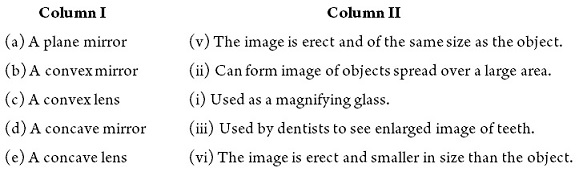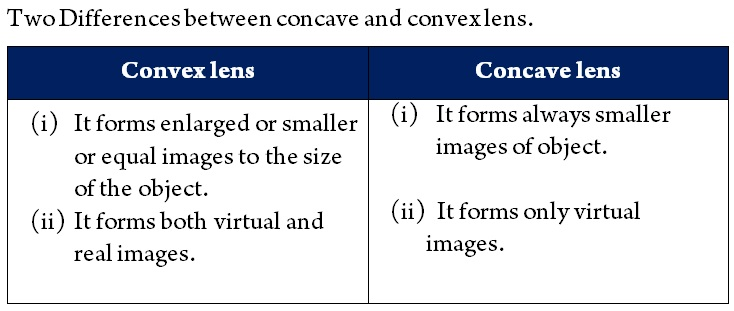Study Materials: ncert solutions

Our ncert solutions for Solutions 15. Light - NCERT Exercise | Class 7 Science - Toppers Study is the best material for English Medium students cbse board and other state boards students.

Solutions ⇒ Class 7th ⇒ Science ⇒ 15. Light

# Solutions 15. Light - NCERT Exercise | Class 7 Science - Toppers Study

Topper Study classes prepares ncert solutions on practical base problems and comes out with the best result that helps the students and teachers as well as tutors and so many ecademic coaching classes that they need in practical life. Our ncert solutions for Solutions 15. Light - NCERT Exercise | Class 7 Science - Toppers Study is the best material for English Medium students cbse board and other state boards students.

## Solutions 15. Light - NCERT Exercise | Class 7 Science - Toppers Study

CBSE board students who preparing for class 7 ncert solutions maths and Science solved exercise chapter 15. Light available and this helps in upcoming exams 2023-2024.

### You can Find Science solution Class 7 Chapter 15. Light

• All Chapter review quick revision notes for chapter 15. Light Class 7
• NCERT Solutions And Textual questions Answers Class 7 Science
• Extra NCERT Book questions Answers Class 7 Science
• Importatnt key points with additional Assignment and questions bank solved.

Chapter 15 Science class 7

### NCERT Exercise class 7 Science Chapter 15. Light

• Solutions 15. Light - NCERT Exercise | Class 7 Science - Toppers Study
• Class 7 Ncert Solutions
• Solution Chapter 15. Light Class 7
• Solutions Class 7
• Chapter 15. Light NCERT Exercise Class 7

## Exercise

Q1. Fill in the blanks:

(a) An image that cannot be obtained on a screen is called ____________.

(b) Image formed by a convex __________ is always virtual and smaller in size.

(c) An image formed by a __________ mirror is always of the same size as that of the object.

(d) An image which can be obtained on a screen is called a _________ image.

(e) An image formed by a concave ___________ cannot be obtained on a screen.

(i) Vertual image

(ii)  miror

(iii) plane

(iv) real

(v) lens

Q2. Mark ‘T’ if the statement is true and ‘F’ if it is false:

(a) We can obtain an enlarged and erect image by a convex mirror. (T/F)

(b) A concave lens always form a virtual image. (T/F)

(c) We can obtain a real, enlarged and inverted image by a concave mirror. (T/F)

(d) A real image cannot be obtained on a screen. (T/F)

(e) A concave mirror always form a real image. (T/F)

(a) False

(b) True

(c) True

(d) False

(e) False

Q3. Match the items given in Column I with one or more items of Column II.

Column I             Column II

(a) A plane mirror    (i) Used as a magnifying glass.

(b) A convex mirror  (ii) Can form image of objects spread over a large                         area.

(c) A convex lens    (iii) Used by dentists to see enlarged image of                              teeth.

(d) A concave mirror (iv) The image is always inverted and magnified.

(e) A concave lens   (v) The image is erect and of the same size as                            the object.

(vi) The image is erect and smaller in size than                            the object.Q4. State the characteristics of the image formed by a plane mirror.

Characteristics of the image formed by a plane mirror are as follows:
(i) Images are virtual and erect.
(ii) it's position is behind the mirror.
(iii) Size of image is equal to size of object.
(iv) Laterally inverted image (image of left side visible on right side).
(v) Distance of image behind the mirror is equal to distance of object in front of mirror.

Q5. Find out the letters of English alphabet or any other language known to you in which the image formed in a plane mirror appears exactly like the letter itself. Discuss your findings.

The letters are A, H, I, M, O, T, U, V, W and X which form same image as the letter is. These letters are laterally symmetrical.

Q6. What is a virtual image? Give one situation where a virtual image is formed.

Answer: The image, which cannot be obtained on a screen, is called virtual image. The images formed by plane mirror, convex mirror and concave lens are always virtual in nature.

Q7. State two differences between a convex and a concave lens.Q8. Give one use each of a concave and a convex mirror.

(i) Concave mirror is used by dentist, headlights in vehicle, As reflector in a torch, etc.
(ii) Convex mirror is used in rear view mirrors.

Q9. Which type of mirror can form a real image?

Answer: Cocave Mirror forms a real image.

Q10. Which type of lens forms always a virtual image? Choose the correct option in questions 11–13

Concave lens.

Q11. A virtual image larger than the object can be produced by a

(i) concave lens  (ii) concave mirror  (iii) convex mirror  (iv) plane mirror

Q12. David is observing his image in a plane mirror. The distance between the mirror and his image is 4 m. If he moves 1 m towards the mirror, then the distance between David and his image will be

(i) 3 m       (ii) 5 m        (iii) 6 m        (iv) 8 m

Q13. The rear view mirror of a car is a plane mirror. A driver is reversing his car at a speed of 2 m/s. The driver sees in his rear view mirror the image of a truck parked behind his car. The speed at which the image of the truck appears to approach the driver will be

(i) 1 m/s      (ii) 2 m/s       (iii) 4 m/s      (iv) 8 m/s